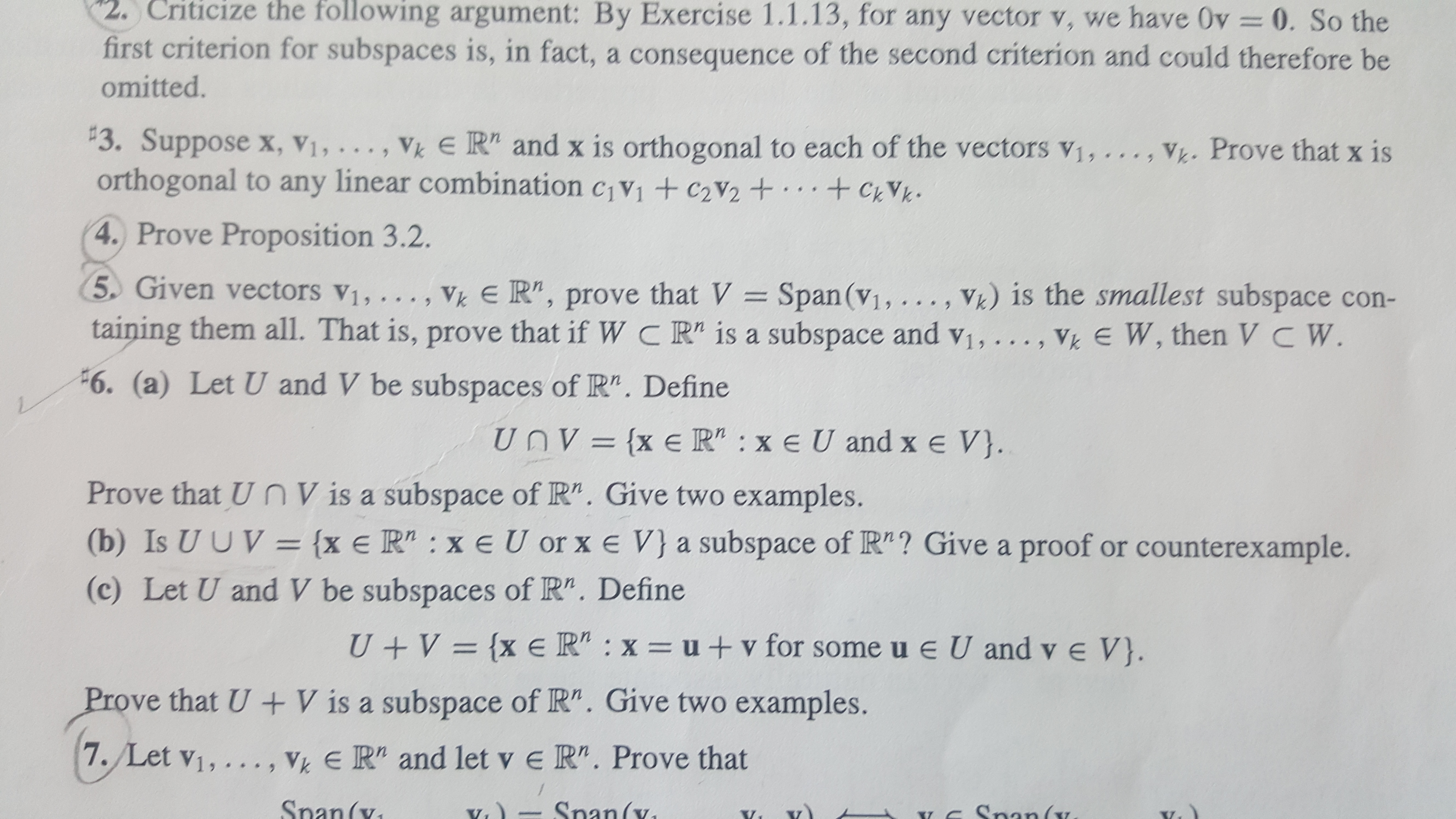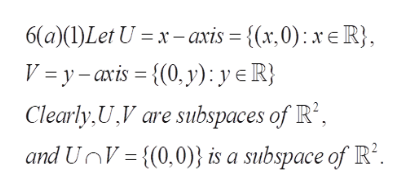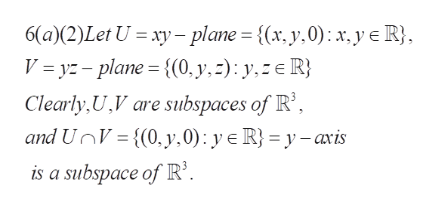# 2. Criticize the following argument: By Exercise 1.1.13, for any vector v, we have Ov 0. So thefirst criterion for subspaces is, in fact, a consequence of the second criterion and could therefore beomitted.3. Suppose x, Vi, . .,orthogonal to any linear combination civi +C2V2 +.+Vk E R" and x is orthogonal to each of the vectors v1,..Vh. Prove that x is,CVks *4. Prove Proposition 3.2.5 Given vectors v1, ..V) is the smallest subspace con-Vk E R", prove that V = Span (v1, ..taining them all. That is, prove that if W C R" is a subspace and vi, ..., Vk E W, then VC W>.6. (a) Let U and V be subspaces of R". DefineUnV={x e R" : xe U and x e V}.Prove that U nVis a subspace of R". Give two examples.(b) Is U UV= {x e R" : x eU or x e V}a subspace of R"? Give a proof or counterexample.(c) Let U and V be subspaces of R". Define{x eR" : x = u + v for some u e U and v e V}.U VProve that U + V is a subspace of R". Give two examples.VkER" and let v e R". Prove that7. Let v1, ... ,Snan(vSnan(v(xSnaY

Question
5 views

-For 6a and 6c. I have done the proof. Please only give me the two examples for each.

Thank you very much!help_outlineImage Transcriptionclose2. Criticize the following argument: By Exercise 1.1.13, for any vector v, we have Ov 0. So the first criterion for subspaces is, in fact, a consequence of the second criterion and could therefore be omitted. 3. Suppose x, Vi, . ., orthogonal to any linear combination civi +C2V2 +.+ Vk E R" and x is orthogonal to each of the vectors v1,.. Vh. Prove that x is , CVk s * 4. Prove Proposition 3.2. 5 Given vectors v1, .. V) is the smallest subspace con- Vk E R", prove that V = Span (v1, .. taining them all. That is, prove that if W C R" is a subspace and vi, ..., Vk E W, then VC W > . 6. (a) Let U and V be subspaces of R". Define UnV={x e R" : xe U and x e V}. Prove that U nVis a subspace of R". Give two examples. (b) Is U UV= {x e R" : x eU or x e V}a subspace of R"? Give a proof or counterexample. (c) Let U and V be subspaces of R". Define {x eR" : x = u + v for some u e U and v e V}. U V Prove that U + V is a subspace of R". Give two examples. VkER" and let v e R". Prove that 7. Let v1, ... , Snan(v Snan(v (x Sna Y fullscreen
check_circle

star
star
star
star
star
1 Rating
Step 1

To give examples for 6(a), 6(c) and counterexamples for 6 (b)

Step 2

6(a) Example 1: Here the intersection of the two subspaces is the 0 vectors subspace.help_outlineImage Transcriptionclose6(a)(I)Let U xaxis = {(x,0) x R} V = y-axis {(0, y): y eR} Clearly, U,V are subspaces of R2 and UnV{(0,0) is a subspace of R2 fullscreen
Step 3

6(a) Example 2: Here , the intersection of the subspaces ...help_outlineImage Transcriptionclose6(a (2)Let U xy-plane = {(x, y.0): x,y e R} V = yz-plane {(0, y,=): y, R} Clearly,U,V are subspaces of R', and UnV{(0, y,0): y e R} = y-avis is a subspace of R'. fullscreen

### Want to see the full answer?

See Solution

#### Want to see this answer and more?

Solutions are written by subject experts who are available 24/7. Questions are typically answered within 1 hour.*

See Solution
*Response times may vary by subject and question.
Tagged in

### Math